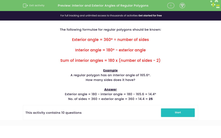# Find the Interior and Exterior Angles of Regular Polygons

In this worksheet, students will apply their knowledge of formulae to calculate the interior and exterior angles of regular polygons.Key stage:  KS 3

Curriculum topic:   Geometry and Measures

Curriculum subtopic:   Derive and Illustrate Properties of Plane Figures

Popular topics:   Angles worksheets, Geometry worksheets

Difficulty level:#### Worksheet Overview

In this activity, we will be looking at regular polygons.

A regular polygon has sides that are all the same length and angles that are all equal.

As in this regular octagon below:The following formulae for regular polygons should be known:

Exterior angle = 360º ÷ number of sides

So with the octagon above, the exterior angle is 360 ÷ 8 = 45º

Interior angle = 180º - exterior angle

So with the octagon, the interior angle is 180 - 45 = 135º

Sum of interior angles = 180 x (number of sides - 2)

So with the octagon, the sum of the interior angles is 180 x (8 - 2) = 180 x 6 = 1,080º

Example

A regular polygon has an interior angle of 165.6º

How many sides does it have?

Exterior angle = 180 - interior angle = 180 - 165.6 = 14.4º

Number of sides = 360 ÷ exterior angle = 360 ÷ 14.4 = 25

So this regular polygon must have 25 sides.

Let's have a go at some questions now.

### What is EdPlace?

We're your National Curriculum aligned online education content provider helping each child succeed in English, maths and science from year 1 to GCSE. With an EdPlace account you’ll be able to track and measure progress, helping each child achieve their best. We build confidence and attainment by personalising each child’s learning at a level that suits them.

Get started